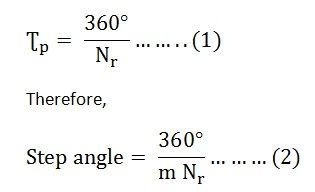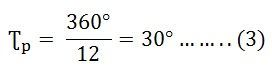# Multi Stack Variable Reluctance Stepper Motor

A Multi Stack or m stack variable reluctance stepper motor is made up of m identical single stack variable reluctance motor. The rotor is mounted on a single shaft. The stator and rotor of the Multi Stack Variable motor have the same number of poles and hence, the same pole pitch.

All the stator poles are aligned in a Multi-Stack motor. But the rotor poles are displaced by 1/m of the pole pitch angle from each other. The stator windings of each stack form one phase as the stator pole windings are excited simultaneously. Thus, the number of phases and the number of stacks are the same.

Consider the cross-sectional view of the three stack motor parallel to the shaft is shown below:There are 12 stator and rotor poles in each stack. The pole pitch for the 12 pole rotor is 30, and the step angle or the rotor pole teeth are displaced by 10 degrees from each other. The calculation is shown below:

Let Nr be the number of rotor teeth and m be the number of stacks or phases.

Hence, tooth pitch is represented by the equation shown below:As there are 12 poles in the stator and rotor, thus the value of Nr = 12. Now, putting the value of Nr in the equation (1) we get,The value of m = 3. Therefore, the step angle will be calculated by putting the value of m in equation (2)When the phase winding A is excited the rotor teeth of stack A is aligned with the stator teeth as shown in the figure below:When phase A is de-energized, and phase B is excited, rotor teeth of stack B are aligned with the stator teeth. The rotor movement is about 10 degrees in the anticlockwise direction. The motor moves one step which is equal to ½ of the pole pitch due to a change of excitation from stack A to stack B. The figure below shows the position of the stator and rotor teeth when phase B is excited.Similarly, now phase B is de-energized, and phase C is excited. The rotor moves another step of 1/3 of the pole pitch in the anticlockwise direction. Again, another change in the excitation of the rotor takes place, and the stator and rotor teeth align it with stack A. However, during this whole process (A – B – C – A ) the rotor has moved one rotor tooth pitch.

Multi Stack Variable Reluctance Stepper Motors are widely used to obtain smaller step angles in the range of 2 to 15 degrees. Both the Variable reluctance motor Single Stack and Multi Stack types have a high torque to inertia ratio.